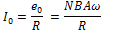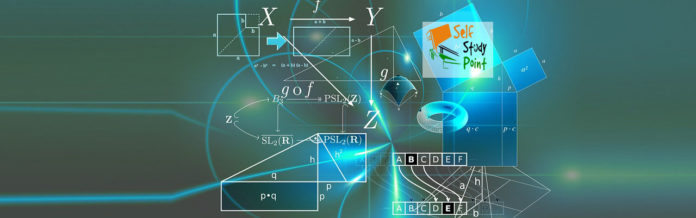### AC Generator:

AC generator is the one which produces a current that alternates or changes its direction regularly after a fixed interval of time, i.e., it is a device which converts mechanical energy into the alternating form of electrical energy.

### The principle of AC Generator:

It is based on the phenomenon of electromagnetic induction–when the coil is rotated with a constant angular velocity W in a uniform magnetic field B, then the magnetic flux linked with the coil changes and an alternating current is induced in the coil.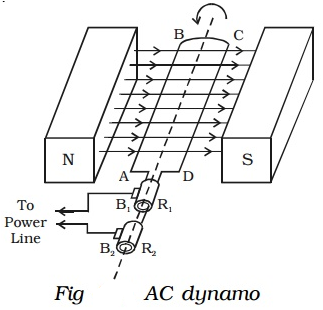### Construction of AC Generator:

It consists of:

(1)    Armature: It is a coil of large no. of turns of copper wire wound over an iron core. (because iron has high permeability). The coil is rotated about its axis.
(2)    Field Magnets: North and south are the pole pieces of a strong magnet in which the coil is rotated. The axis of the coil is perpendicular to the magnetic field.
(3)    Rings (R1 and R2): (also called slip rings): These are the two hollow metallic rings which are connected to the ends of the coil. These rings rotate with the rotation of coil.
(4)    Brushes (B1 and B2): These are the two metal plates or carbon rods. They are fixed and kept in contact with R1 and R2. The purpose of the brushes is to pass current from the armature to the external load resistance.

### Theory of Working of AC Generator:

Suppose that the plane of the coil is such that its axis is perpendicular to the magnetic field. As the coil is rotated clockwise, the side AB of the coil moves upward and the other side CD moves downwards. The flux in the coil changes, the current is induced, the direction of the current in the side AB will be outward according to Fleming’s left-hand rule. In the next half cycle, AB moves downward and CD moves upward. Now, the direction of current induced in AB is inward according to Fleming’s left-hand rule. Thus, alternating current is induced in the coil in one complete cycle which flows from the brushes B1 and B2 to the external load resistance such that an alternating voltage is developed across RL.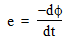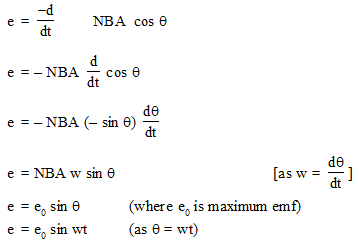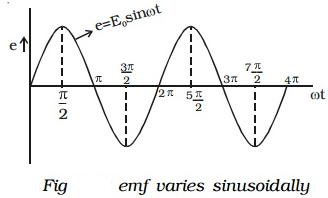Maximum induced emf,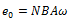When a load of resistance R is connected across the terminals, a current I flows in the external circuit.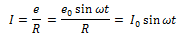where I0 = e0/R , both current and voltage vary sinusoidally with time.

Maximum current,